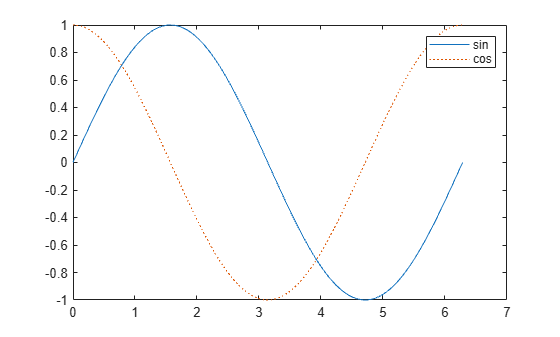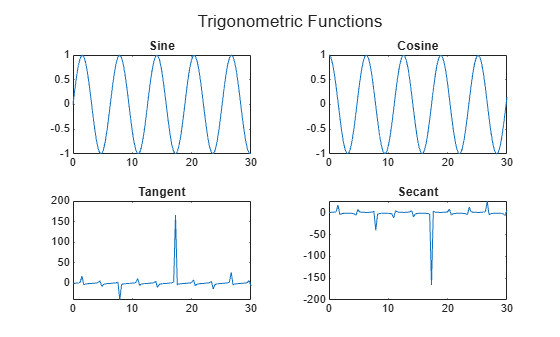# 2-D and 3-D Plots

### Line Plots

To create two-dimensional line plots, use the `plot` function. For example, plot the sine function over a linearly spaced vector of values from 0 to $2\pi$:

```x = linspace(0,2*pi); y = sin(x); plot(x,y)```You can label the axes and add a title.

```xlabel("x") ylabel("sin(x)") title("Plot of the Sine Function")```By adding a third input argument to the `plot` function, you can plot the same variables using a red dashed line.

`plot(x,y,"r--")``"r--"` is a line specification. Each specification can include characters for the line color, style, and marker. A marker is a symbol that appears at each plotted data point, such as a `+`, `o`, or `*`. For example, "`g:*"` requests a dotted green line with `*` markers.

Notice that the titles and labels that you defined for the first plot are no longer in the current figure window. By default, MATLAB® clears the figure each time you call a plotting function, resetting the axes and other elements to prepare the new plot.

To add plots to an existing figure, use `hold on`. Until you use `hold off` or close the window, all plots appear in the current figure window.

```x = linspace(0,2*pi); y = sin(x); plot(x,y) hold on y2 = cos(x); plot(x,y2,":") legend("sin","cos") hold off```### 3-D Plots

Three-dimensional plots typically display a surface defined by a function in two variables, $z=f\left(x,y\right)$. For instance, calculate $z=x{e}^{-{x}^{2}-{y}^{2}}$ given row and column vectors `x` and `y` with 20 points each in the range [-2,2].

```x = linspace(-2,2,20); y = x'; z = x .* exp(-x.^2 - y.^2);```

Then, create a surface plot.

`surf(x,y,z)`Both the `surf` function and its companion `mesh` display surfaces in three dimensions. `surf` displays both the connecting lines and the faces of the surface in color. `mesh` produces wireframe surfaces that color only the connecting lines.

### Multiple Plots

You can display multiple plots in different parts of the same window using either `tiledlayout` or `subplot`.

The `tiledlayout` function was introduced in R2019b and provides more control over labels and spacing than `subplot`. For example, create a 2-by-2 layout within a figure window. Then, call `nexttile` each time you want a plot to appear in the next region.

```t = tiledlayout(2,2); title(t,"Trigonometric Functions") x = linspace(0,30); nexttile plot(x,sin(x)) title("Sine") nexttile plot(x,cos(x)) title("Cosine") nexttile plot(x,tan(x)) title("Tangent") nexttile plot(x,sec(x)) title("Secant")```If you are using a release earlier than R2019b, see `subplot`.